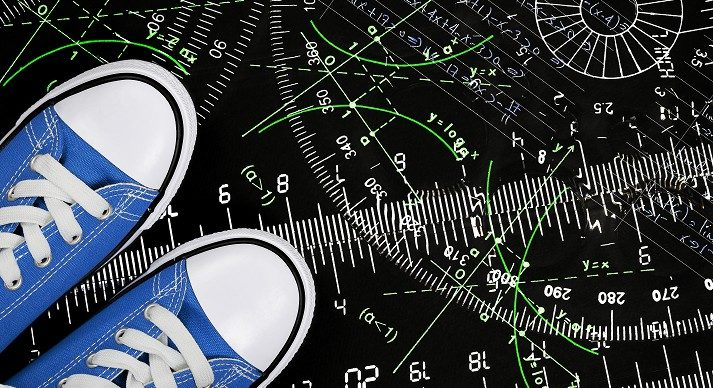High School Math IV-SECourse Overview

High School Math IV-SE provides Special Ed students with a basic foundation in math. Some of the major focus areas of this course include integers, decimals, and fractions. This course focuses on the concepts most important for these students, digging in deeper than the regular course to provide the additional instruction needed. Acellus High School Math IV-SE is taught by Acellus Instructor Kelly Frogge.This course was developed by the International Academy of Science. Learn More

Scope and Sequence

Unit 1 In this unit students study integers. They explore comparison, addition, subtraction, multiplication and division. They learn about opposites, absolute value, using a chip model and a number line, and order of operations. Unit 2 In this unit students focus on decimals. They study interpretation, comparison, estimation, and rounding. They practice addition, subtraction, multiplication, and division. They explore converting percents to decimals and vice versa. Unit 3 In this unit students investigate fractions and continue their study of decimals. They discover greatest common factors, least common multiples, and reducing fractions. They learn to convert fractions to mixed numbers, terminating decimals, and repeating decimals, and vice versa. They explore repeating and terminating decimals, as well as ordering rational numbers. Unit 4 Continuing their study of fractions, in this unit students explore finding a common denominator, as well as addition, subtraction, multiplication, and division of simple fractions, mixed numbers, mixed numbers with regrouping, rational numbers, and negative fractions. Following this unit students are presented with the Mid-term Review and Exam. Unit 5 In this unit students focus on algebraic expressions. They explore the difference between expressions and equations. They learn to evaluate, simplify, expand, and factor algebraic expressions, all working with integers and with rational numbers. They also investigate adding and subtracting rational co-efficients and recognizing equivalent expressions. Unit 6 In this unit students explore equations. They learn to write equations and inequalities, and to graph inequalities, as well as to sovle inequalities and one- and two-step equations. They also explore equations with rational numbers, and equations with variables on both sides. Unit 7 In this unit students focus on angles. They study adjacent, complementary, supplementary, reflex, vertex-sharing, and vertical angles. They also investigate how triangles are related to angles, as well as drawing triangles. Unit 8 In this lesson students focus on geometry. They study circles, including vocabulary, circumference, radius, and diameter. They learn about finding area, including area of circles, parallelograms, triangles, and trapezoids. Unit 9 In this unit students continue their study of geometry. They learn to identify three-dimensional figures and nets. They explore cross sections. They gain understanding of the surface area and volume of prisms, pyramids and cylinders. Unit 10 In this unit students study ratios and proportion. They also learn about unit rate and simple interest. Unit 11 In this unit students focus on statistics. They learn about mean, mode, median, and range. They investigate box and whisker plots, random sampling, and comparing data sets. Following this unit students are presented with the Final Review and Exam.